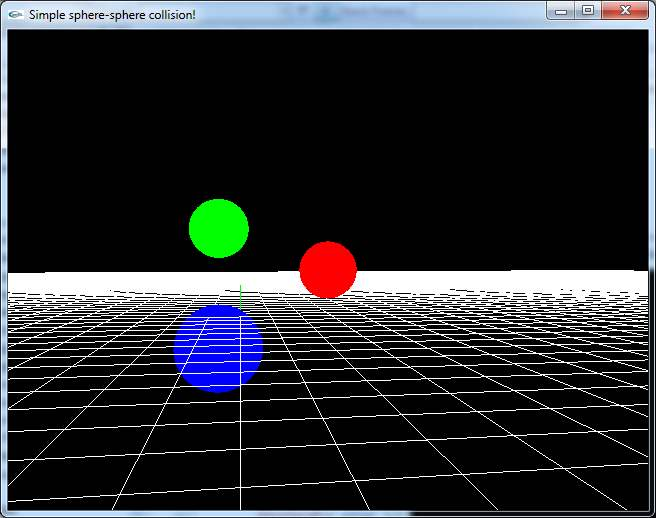# Simple Sphere-Sphere Collision Detection and Collision Response

By , last updated September 25, 2019

## Collision Detection

To determine if two spheres are colliding, we take the sum of the radiuses and compare it with the length from the centers of the spheres. If the length is smaller than the sum of the radiuses, we have a collision.

The difference vector (the length is the distance between those two spheres):$vec{d} = s1.pos - s2.pos$

Then the length is computed:$distance = vec{d}.length = sqrt{vec{d}.x^2 + vec{d}.y^2 + vec{d}.z^2}$$sumradius = s1.radius + s2.radius$

If distance < sumradius the we have a collision to take care of. This is an example of an elastic collision detection. More advanced algorithm includes calculations of collision time and direction.

## Collision Response

This is a little bit trickier, but with some basics explained, it should be pretty straight forward.

First, find the vector which will serve as a basis vector (x-axis), in an arbitrary direction. It has to be normalized to get realistic results.$vec{x} = s1.pos - s2.pos$$vec{x}.normalize()$

Then we calculate the x-direction velocity vector and the perpendicular y-vector.$vec{v1} = s1.vel$$x1 = x.dot(vec{v1})$$vec{v1x} = vec{x} * x1$$vec{v1y} = vec{v1} - vec{v1x}$$m1 = s1.mass$

Same procedure for the other sphere.$vec{x} = vec{x}*-1$$vec{v2} = s2.vel$$x2 = vec{x}.dot(vec{v2})$$vec{v2x} = vec{x} * x2$$vec{v2y} = vec{v2} - vec{v2x}$$m2 = s2.mass$

Then we mix and play around with some of Newton’s laws to obtain a formula for the speed (in vector format) after the collision.$s1.vel = vec{v1x}{(m1-m2)/(m1+m2)} + vec{v2x}{(2*m2)/(m1+m2)} + vec{v1y}$$s2.vel = vec{v1x}{(2*m1)/(m1+m2)} + vec{v2x}{(m2-m1)/(m1+m2)} + vec{v2y}$

And it actually works! 🙂

## Sample application:Professional Software Developer, doing mostly C++. Connect with Kent on Twitter.